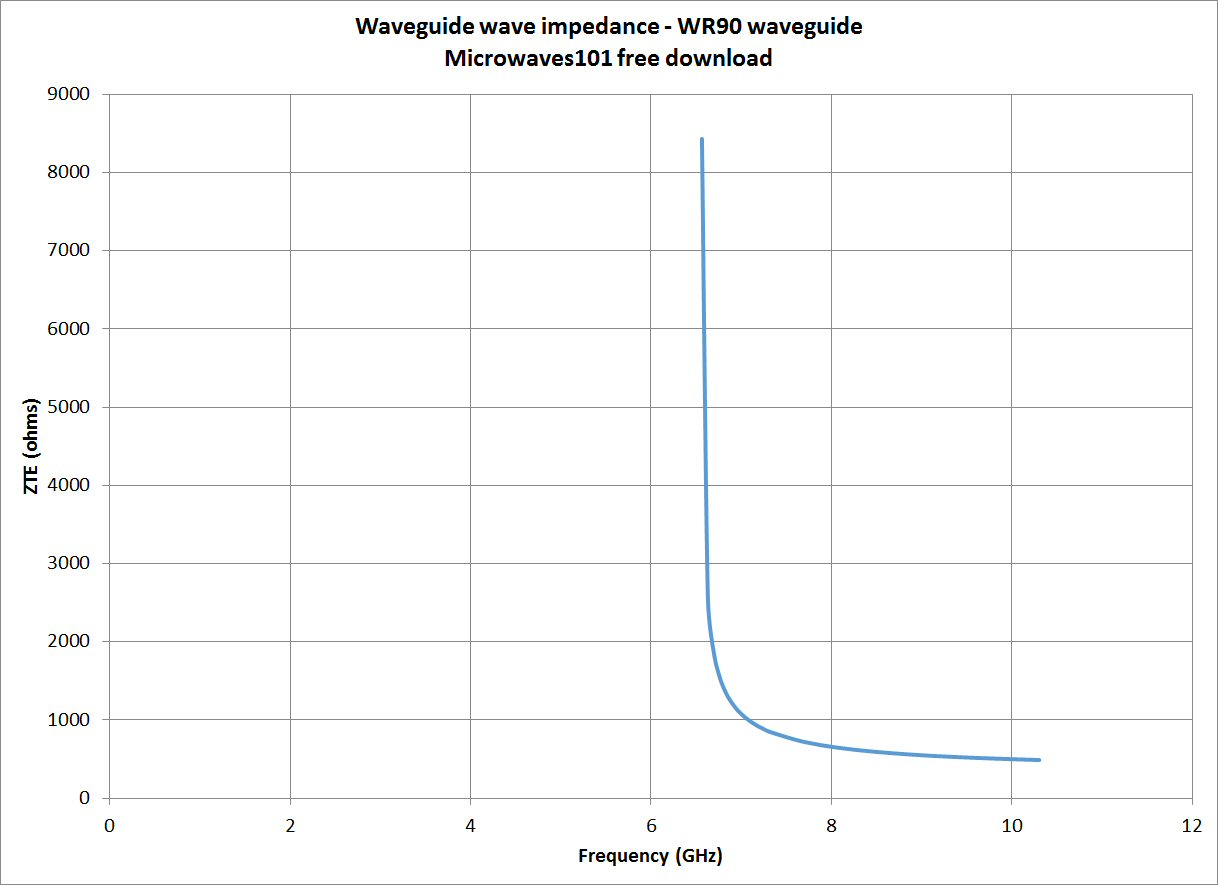# Waveguide wave impedance

Most coax circuits are 50 ohms or 75 ohms.  What about waveguide? Does it even have an impedance?

Yes, it does, it is called the "wave impedance".  There are at least two ways to calculate it. For reference we suggest Pozar's "Microwave Engineering", pages 96 and 97.

For transverse electric waves,

ZTE=ωμ/β

For transverse magnetic waves,

ZTM=β/(ωε)

However, considering waveguide wave impedance as a true impedance, similar to a transmission line, will give you a headache. Here is an appropriate definition of wave impedance sent in by one of our readers:

I think it is a topic worth explaining, but I am not quite sure wave impedance is the most useful definition. For example, in coax transmission lines, wave impedance is independent of the dimensions, contrary to what one would except from an impedance.

A useful definition would be the ratio of the "voltage" calculated by integration of the electric field on the center of the waveguide divided by the "total current" obtained by integration of the magnetic field on the top wall. This definition is also nice for substrate integrated waveguide (SIW) to microstrip transition problems.

Here's a plot of ZTE for WR-90, which is used at X-band. In-band, it is on the order of 500 ohms but has a downward slope.  As cutoff is reached (below band), wave impedance goes to infinity.  We generated this plot on our Waveguide Loss Excel spreadsheet which you can get for free in our download area.  Note that when you calculate wave impedance using our spreadsheet you will find it is NOT a function of the height of the guide.Both impedance terms are functions of frequency and mode.  As a Microwaves101 rule of thumb, waveguide wave impedance is approximately 500 ohms for standard rectangular waveguide. our Waveguide loss spreadsheet, loccated in the download area, calculates wave impedance for you.

Author : Unknown Editor